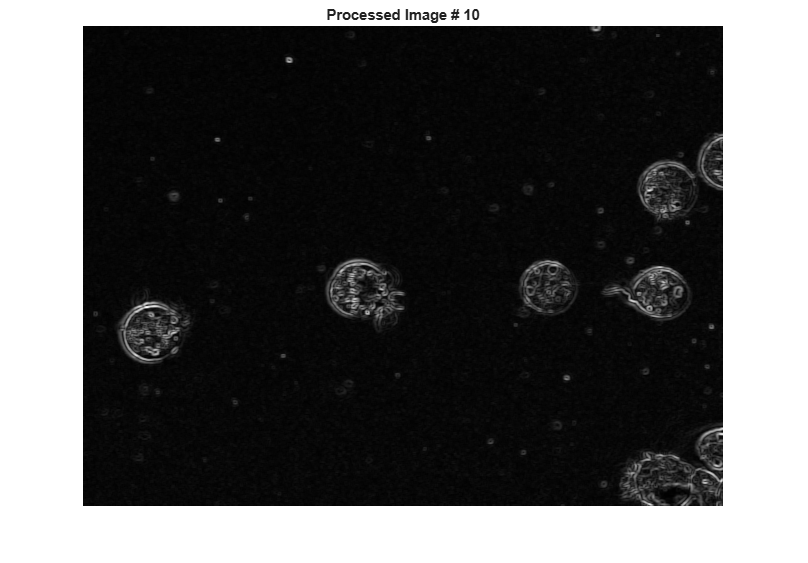# Perform an Operation on a Sequence of Images

This example shows how to perform an operation on a sequence of images. The example creates an array of images and passes the entire array to the `stdfilt` function to perform standard deviation filtering on each image in the sequence.

Create an array of file names.

```fileFolder = fullfile(matlabroot,'toolbox','images','imdata'); dirOutput = dir(fullfile(fileFolder,'AT3_1m4_*.tif')); fileNames = {dirOutput.name}' numFrames = numel(fileNames) ```
```fileNames = 10x1 cell array {'AT3_1m4_01.tif'} {'AT3_1m4_02.tif'} {'AT3_1m4_03.tif'} {'AT3_1m4_04.tif'} {'AT3_1m4_05.tif'} {'AT3_1m4_06.tif'} {'AT3_1m4_07.tif'} {'AT3_1m4_08.tif'} {'AT3_1m4_09.tif'} {'AT3_1m4_10.tif'} numFrames = 10 ```

Preallocate an m -by- n -by- p array and read images into the array.

```I = imread(fileNames{1}); sequence = zeros([size(I) numFrames],class(I)); sequence(:,:,1) = I; for p = 2:numFrames sequence(:,:,p) = imread(fileNames{p}); end ```

Process each image in the sequence, performing standard deviation filtering. Note that, to use `stdfilt` with an image sequence, you must specify the `nhood` argument, passing a 2-D neighborhood.

```sequenceNew = stdfilt(sequence,ones(3)); ```

View each input image followed by its processed image.

```figure; for k = 1:numFrames imshow(sequence(:,:,k)); title(sprintf('Original Image # %d',k)); pause(1); imshow(sequenceNew(:,:,k),[]); title(sprintf('Processed Image # %d',k)); pause(1); end ```